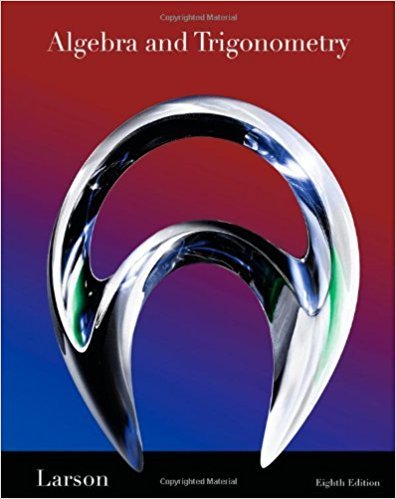×
×

# Solutions for Chapter 7.1: Using Fundamental Identities## Full solutions for Algebra and Trigonometry | 8th Edition

ISBN: 9781439048474Solutions for Chapter 7.1: Using Fundamental Identities

Solutions for Chapter 7.1
4 5 0 236 Reviews
13
2
##### ISBN: 9781439048474

This textbook survival guide was created for the textbook: Algebra and Trigonometry, edition: 8. This expansive textbook survival guide covers the following chapters and their solutions. Chapter 7.1: Using Fundamental Identities includes 142 full step-by-step solutions. Algebra and Trigonometry was written by and is associated to the ISBN: 9781439048474. Since 142 problems in chapter 7.1: Using Fundamental Identities have been answered, more than 51738 students have viewed full step-by-step solutions from this chapter.

Key Math Terms and definitions covered in this textbook
• Diagonal matrix D.

dij = 0 if i #- j. Block-diagonal: zero outside square blocks Du.

• Elimination.

A sequence of row operations that reduces A to an upper triangular U or to the reduced form R = rref(A). Then A = LU with multipliers eO in L, or P A = L U with row exchanges in P, or E A = R with an invertible E.

• Fast Fourier Transform (FFT).

A factorization of the Fourier matrix Fn into e = log2 n matrices Si times a permutation. Each Si needs only nl2 multiplications, so Fnx and Fn-1c can be computed with ne/2 multiplications. Revolutionary.

• Fourier matrix F.

Entries Fjk = e21Cijk/n give orthogonal columns FT F = nI. Then y = Fe is the (inverse) Discrete Fourier Transform Y j = L cke21Cijk/n.

• Lucas numbers

Ln = 2,J, 3, 4, ... satisfy Ln = L n- l +Ln- 2 = A1 +A~, with AI, A2 = (1 ± -/5)/2 from the Fibonacci matrix U~]' Compare Lo = 2 with Fo = O.

• Markov matrix M.

All mij > 0 and each column sum is 1. Largest eigenvalue A = 1. If mij > 0, the columns of Mk approach the steady state eigenvector M s = s > O.

• Matrix multiplication AB.

The i, j entry of AB is (row i of A)·(column j of B) = L aikbkj. By columns: Column j of AB = A times column j of B. By rows: row i of A multiplies B. Columns times rows: AB = sum of (column k)(row k). All these equivalent definitions come from the rule that A B times x equals A times B x .

• Multiplicities AM and G M.

The algebraic multiplicity A M of A is the number of times A appears as a root of det(A - AI) = O. The geometric multiplicity GM is the number of independent eigenvectors for A (= dimension of the eigenspace).

• Nilpotent matrix N.

Some power of N is the zero matrix, N k = o. The only eigenvalue is A = 0 (repeated n times). Examples: triangular matrices with zero diagonal.

• Nullspace matrix N.

The columns of N are the n - r special solutions to As = O.

• Positive definite matrix A.

Symmetric matrix with positive eigenvalues and positive pivots. Definition: x T Ax > 0 unless x = O. Then A = LDLT with diag(D» O.

• Projection p = a(aTblaTa) onto the line through a.

P = aaT laTa has rank l.

• Rank r (A)

= number of pivots = dimension of column space = dimension of row space.

• Saddle point of I(x}, ... ,xn ).

A point where the first derivatives of I are zero and the second derivative matrix (a2 II aXi ax j = Hessian matrix) is indefinite.

• Similar matrices A and B.

Every B = M-I AM has the same eigenvalues as A.

• Standard basis for Rn.

Columns of n by n identity matrix (written i ,j ,k in R3).

• Symmetric matrix A.

The transpose is AT = A, and aU = a ji. A-I is also symmetric.

• Transpose matrix AT.

Entries AL = Ajj. AT is n by In, AT A is square, symmetric, positive semidefinite. The transposes of AB and A-I are BT AT and (AT)-I.

• Unitary matrix UH = U T = U-I.

Orthonormal columns (complex analog of Q).